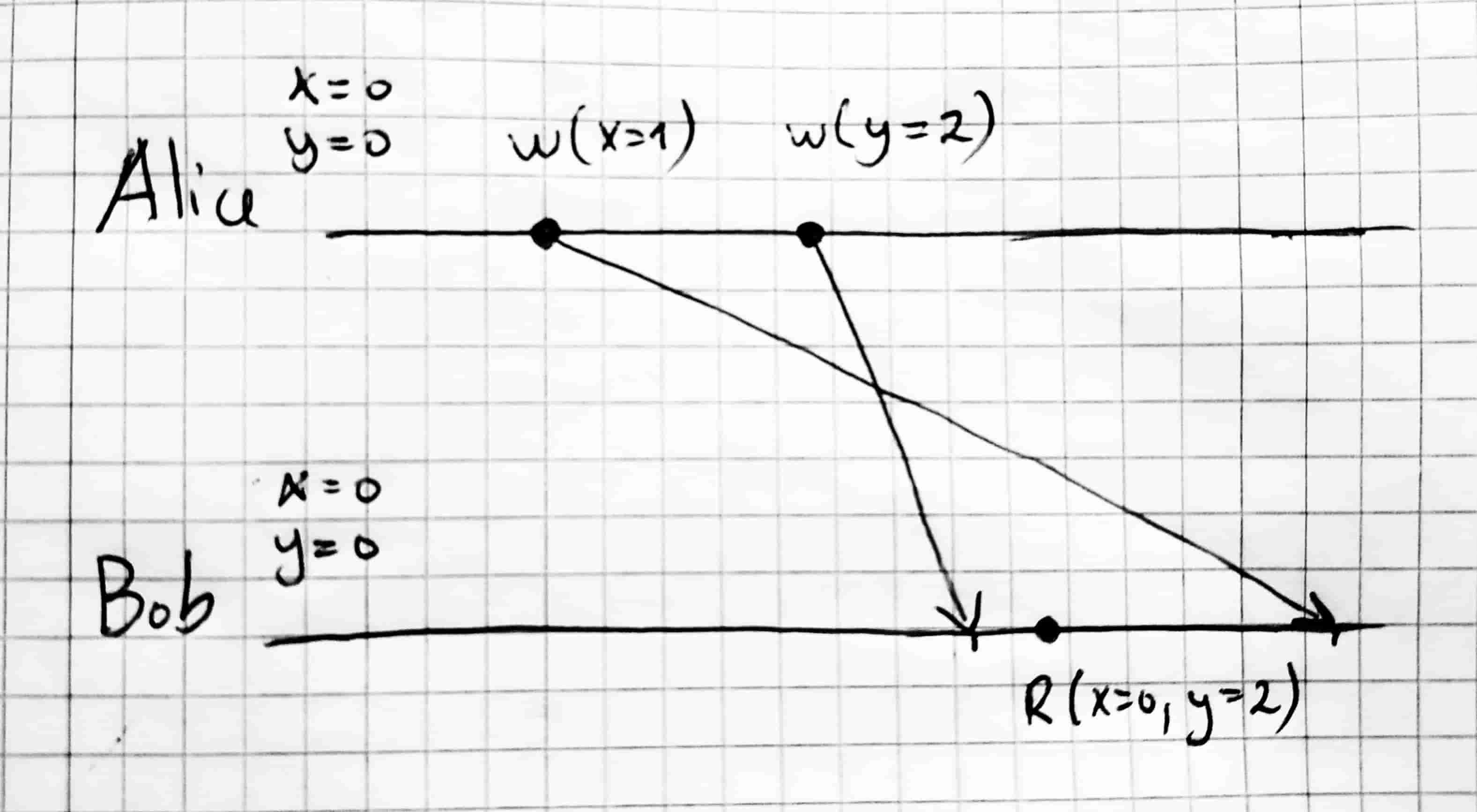Fault-tolerant Causal Stability (part I)

You guessed it! This post shows that 1) causal stability is not fault-tolerant but 2) we can do something about it.

Well, that was the intention, at least. The blog post was becoming too long, so I’ll divide it in (exactly those) two:

• part I: revisits the concepts of causal consistency and causal stability; we’ll also discuss how these two concepts can be implemented in a simple way; we’ll conclude that causal stability is not fault-tolerant
• part II: shows that causal stability can be fault-tolerant and the level of fault-tolerance provided simply depends on the size of the write quorum

Causal Consistency

In simple terms, causal consistency ensures that:

• if operation $o$ causes operation $p$
• then every process in the system will not observe $p$ without observing $o$

In other words, no effect is observed without its cause.

In Figure 1 we have an example where causal consistency is not respected. We start two objects $x$ and $y$ initialized at $0$. Alice writes $x = 1$ and then $y = 2$. At this point, in a causally consistent system, Bob can see:

• $x = 0 \land y = 0$
• $x = 1 \land y = 0$
• $x = 1 \land y = 2$

However, it ended up reading $x = 0 \land y = 2$ (i.e. it saw the second write by Alice without seeing the first), and with that, the run in Figure 1 is not causally consistent.A run that is not causally consistency.

Implementing causal consistency

We can implement a causally consistent system with two simple steps:

• attach to each operation $o$ a set containing all its preceding operations; let’s call this set the dependencies of $o$ and denote it by $\textsf{dep}[o]$
• execute $o$ once all operations in $\textsf{dep}[o]$ have been executed

(Note how this fixes the problem presented in Figure 1.)

Let’s also denote the set of executed operations by $\textsf{Executed}$.

If the process submits operation $o$, then the dependencies of $o$ will be the set of operations that have been executed at that moment, i.e. $\textsf{dep}(o) = \textsf{Executed}$.

A note on concurrency

If operation $o$ causes operation $p$, then $o$ is part of the set of dependencies of $p$:

$$o \in \textsf{dep}(p)$$

However, it can happen that $o$ doesn’t cause $p$ and $p$ doesn’t cause $o$.
When this is the case, we say that $o$ and $p$ are concurrent:

$$o \not \in \textsf{dep}(p) \land p \not \in \textsf{dep}(o)$$

Causal Stability

An operation $o$ is causally stable (denoted by $\textsf{stable}(o)$) if every operation $p$ that will be executed in the system must be caused by $o$:

$$\textsf{stable}(o) \equiv \forall p \cdot p \not \in \textsf{Executed} \implies o \in \textsf{dep}(p)$$

In other words, if $\textsf{stable}(o)$ then every operation will be in the future of $o$.

The concept of causal stability is exploited, for example, by CRDTs (Section 4.1) to perform garbage-collection (Section 7.2).

Getting some intuition with an example

Let’s pause for a bit with an example where we compute the set of stable operations.

In this example we have:

• 3 processes: a, b and c
• 4 operations: $o$, $p$, $q$ and $r$

Let’s say that:

• a submitted $o$ and then $p$
• b saw $o$ and $p$, and then submitted $q$
• c saw $o$ and submitted $r$

So, we have the following $\textsf{Executed}$:

• $\textsf{Executed}_{\textbf{a}} = \{o, p\}$
• $\textsf{Executed}_{\textbf{b}} = \{o, p, q\}$
• $\textsf{Executed}_{\textbf{c}} = \{o, r\}$

Recall that: the set of dependencies of an operation is the set of executed operations at the time the operation is submitted.

So at this point we know that any operation submitted (by any process) will include $o$ as a dependency. This means that $o$ is stable.

We can’t say the same about any of the remaining operations.

Implementing causal stability

Let’s denote the set of all processes in the system by $\mathcal{I}$.

Stability can be detected if we learn1 what’s been executed by each process, i.e. we know $\textsf{Executed}_i$ for every process $i \in \mathcal{I}$.

Then, the set of stable operations is given by the intersection of all $\textsf{Executed}_i$’s:

$$\bigcap_{i \in \mathcal{I}} \textsf{Executed}_i$$

A (second) note on concurrency

Given operation $o$, $\textsf{stable}(o)$ implies that all operations concurrent with $o$ have also been executed.

Let’s see why that is:

• for some operation $p$ be concurrent with $o$, then $o$ can’t be a dependency of $p$: $o \not \in \textsf{dep}(p)$
• however, this contradicts $\textsf{stable}(o)$ that requires that $o$ is a dependency of every operation that will be executed, and in particular, that it is a dependency of $p$: $o \in \textsf{dep}(p)$.

Causal stability is a local property

What’s stable on one process might not (yet) be stable on other processes.

This is because processes compute stability based on what they know others have executed, and different processes might have different views on what’s been executed.

However, if an operation is stable on some process, it will eventually become stable on every process.

(Not-so-fault-tolerant) Causal Stability

At this point, we already know why causal stability is not fault-tolerant.

In order to advance stability, processes need to learn what all processes are executing, and if some process crashes, the set of stable operations will not grow. And that’s not fault-tolerant.

So in part II we’ll try to fix that!

UPDATE: I’ll address the concerns raised in the replies to my tweet in the next blog post.

1. This can be implemented, for example, by having processes periodically broadcasting what they have executed. ^